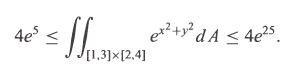# Help with a proof.

## Homework Statement

Hi. I have been given the following question.

Show that this is true:## The Attempt at a Solution

Well I drew out the region and the bounds. I'm pretty sure that it isn't possible to integrate this because no matter the order, I need either an x or a y in there to do a substitution. So I'm guessing that I just have to show in some way that the final integral solution will lie between those two numbers, but I don't know how to approach this.

LCKurtz
Homework Helper
Gold Member
Think about how large and how small the integrand can be on that domain.

Try your luck with a polar transformation if you want to save time on the integral.

I didn't do polar transformations yet.

That domain is a square, but how can i find how large it can be without knowing the integral?

dextercioby
Homework Helper
You can't use a polar transformation, because the domain is a finite rectangle.

Mark44
Mentor
ex2 + y2 will have its largest value at one of the corner points of the region. Similarly, this function will have its smallest value at another of the corner points.

ex2 + y2 will have its largest value at one of the corner points of the region. Similarly, this function will have its smallest value at another of the corner points.

Makes sense, but I'm not sure how i can show that without doing the integral itself.

Mark44
Mentor
You DON'T need to calculate the integral. This problem is basically an extension of the ideas behind approximating an integral using a Riemann sum. Instead of using rectangles to get an upper and lower estimate for the area beneath a curve, here you're using boxes to get upper and lower estimates for the volume beneath a surface.

LCKurtz
Homework Helper
Gold Member
You can't use a polar transformation, because the domain is a finite rectangle.

Actually, to be pedantic, he could use a polar transformation if he had studied that, but it would be pointless and wouldn't help.

@Kuma: Use the theorem if m ≤ f(x,y) ≤ M on a region R then
$$m\cdot Area(R) \le \iint_R f(x,y)\, dydx\le M\cdot Area(R)$$

dextercioby